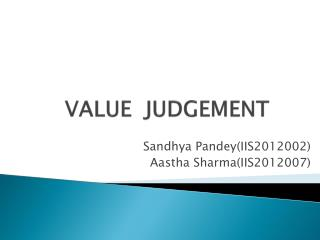DownloadDownload PresentationVALUE JUDGEMENT

# VALUE JUDGEMENT

Télécharger la présentation## VALUE JUDGEMENT

- - - - - - - - - - - - - - - - - - - - - - - - - - - E N D - - - - - - - - - - - - - - - - - - - - - - - - - - -
##### Presentation Transcript

1. VALUE JUDGEMENT SandhyaPandey(IIS2012002) Aastha Sharma(IIS2012007)

2. INTRODUCTION • The value judgment computes the cost, benefit, risk and expected payoff of plans. • It assigns value to objects, events and situations. • It decides what is important or trivial, what is rewarding and punishing, and what degree of confidence to assign to enteries in the world model.

3. It evaluates both the observed state of the world and the predicted results of hypothesized plans by computing their cost risk and benefits. • It computes the probability of correctness and assigns believability and uncertainty parameters to state variables. • Provides the basis for decision making or choosing one action instead of another. • Without VJ any biological creature would soon be destroyed and any artificial intelligent system would soon be disabled by its own inappropriate actions.

4. Functional Relationship Of VJ With Other Modules Of RCS

5. Why do we need VJ? • Since intelligent systems are goal oriented, their functioning requires them to know ,at each step, what is better and what is worse. • We will try and determine how these concepts can fit into a more general paradigm of intelligent system. • To fulfill above requirements of an artificial intelligent system value judgment module is included in its architecture.

6. Working of VJ • VJ module produce evaluation that can be represented as value state variables. • These can be assigned to the attribute list in entity frames of objects, persons, events, situations and reasons of space. • They can also be assigned to the attribute lists of plans and actions in task frames. • Hence VJ provide criteria for decisions about which course of action to take.

7. Value State Variables • Value state variables are used to label entities , tasks and plans as good or bad, costly or inexpensive, as important or trivial, as reliable or uncertain. • These variables can also be used by the BG module for both planning and executing actions.

8. Examples of value state variables 1.Emotions 8.Priorities 2.Drives 9.Goodness & badness 3.Confidence 10.Pleasure and pain 4.Success_observed 11.Success_expected 5.Hope & frustration 12.Comfort 6.Fear 13. Joy 7.Uncertainity 14.Despair

9. Numerical Representation of value state variable • It is possible to assign a real non negative numerical scalar value to each state variable. • This defines the degree or amount of that value assigned to the state variable. • For example, a positive real value assigned by “goodness” defines how good; if e=“goodness” and 0≤e≤10. then e=10 is the “best” evaluation possible.

10. Numerical Representation…. • Some value state-variables can be grouped as conjugate pairs. For example, goodness-badness, pleasure-painand success-fail. • For conjugate pairs, a positive real value means the amount of the good value, and a negative real value means the bad value. • For example; 1. e=“goodness-badness” and -10≤e≤+10. 2. e=“pleasure-pain” and -10≤e≤+10.

11. Mathematical Model of VJ • Values for the state-variables are computed by value judgment functions residing in the modules. • Inputs to VJ modules describe entities, events, situations and states. • VJ functions compute measures of cost, risk and benefit. • VJ outputs are value state-variables.

12. Mathematical model… The VJ mechanism can be defined as a mathematical or logical function of the form E=V(S), Where E is an output vector of value state-variables. V is a value judgment function that computes E given S. S is an input state vector defining conditions in the WM, including the self. The components of S are entity attributes describing states of tasks, objects, events or regions of space. These may be derived either from processed sensory information or from the world model.

13. Mathematical model… • The value function V in the VJ module computes a numerical scalar value for each component of E as a function of the input state vector S. E is a time independent vector. • The component of E may be assigned to attributes in the world model frame of various entities, events or states.

14. Mathematical model with time dependency • If time dependency is included, the function becomes; E(t+dt)=V(S(t)) This may be computed by a set of equation of the form e(j, t+dt)=(k ∑i s(i, t)w(i, j) Where e(j, t) is the value of the jth value state variable in the vector E at time t. s(i, j) is the value of the ith input variable at the time t. W(i, j) is a coefficient, or weight, that defines the contribution of s(i) to e(j).

15. The factor (k d/dt +1) indicates that a value judgment is typically dependent on the temporal derivative of its input variable as well as on their steady state value. • Following example describes how a VJ function might compute the value state-variable “happiness” happiness=(k d/dt+1)(success-expectation +hope-frustration + love-hate +comfort-fear +joy-despair),

16. Example: VJ Module for computing pleasure and pain

17. Thank You